Euler’s EquationThe last equation is a homogeneous linear second order differential equation with constant coefficients: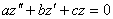where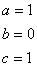For these simple equations, we look for a solution of the formSubstituting these values we get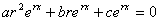Dividing through by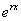gives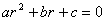which is the characteristic equation of the differential equation.  The solution of this equation can be derived from the quadratic equation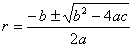Substituting the values givesThe two solutions to the differential equation are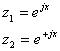And the complete solution is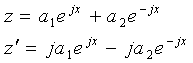Recalling thatSo the final solution is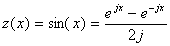Taking the derivativeThis is Euler’s equation!